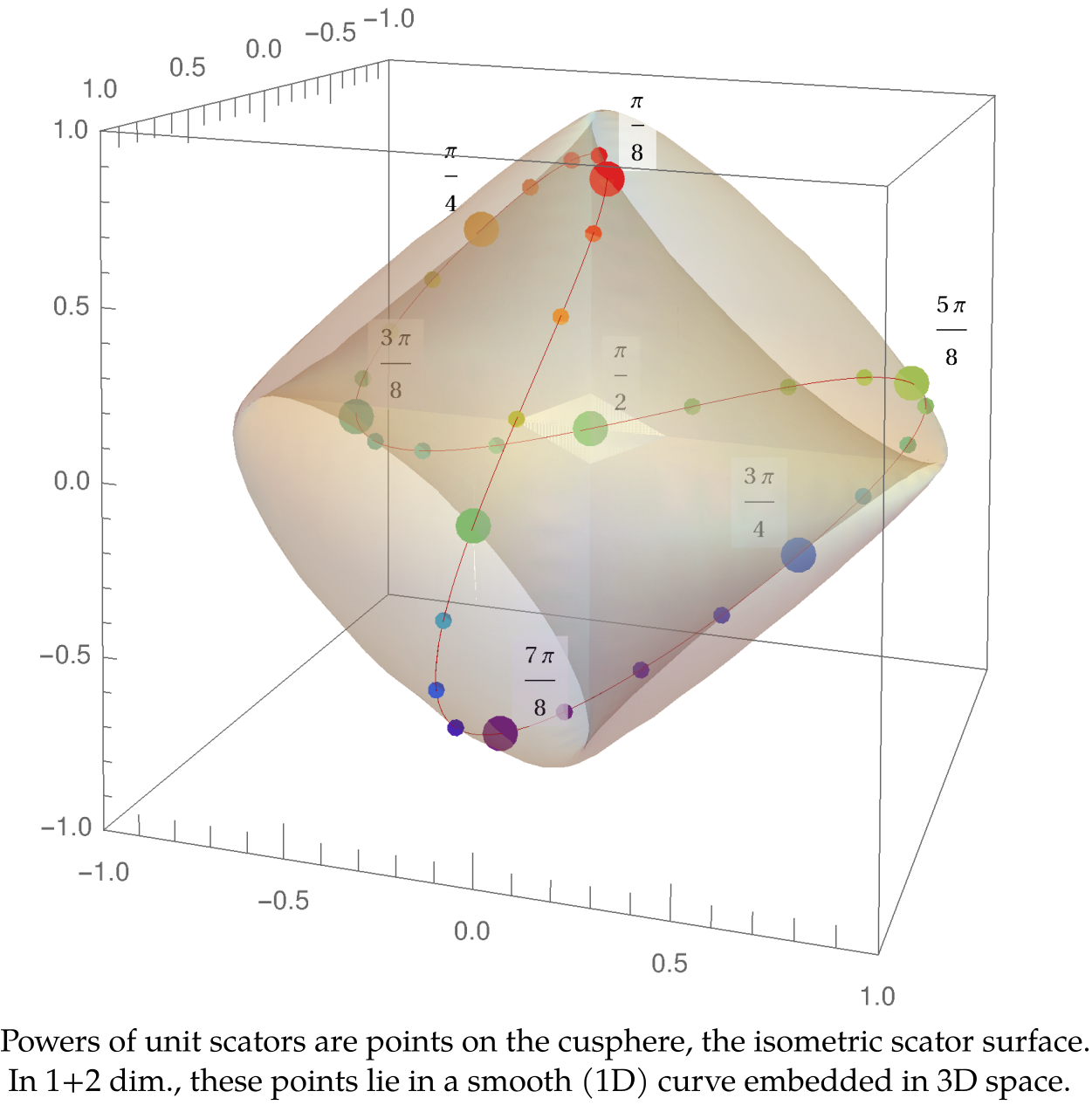Preprint Article Version 1 Preserved in Portico This version is not peer-reviewed

# Powers of Elliptic Scator Numbers

Version 1 : Received: 29 August 2021 / Approved: 31 August 2021 / Online: 31 August 2021 (11:51:03 CEST)

A peer-reviewed article of this Preprint also exists.

Fernandez-Guasti, M. Powers of Elliptic Scator Numbers. Axioms 2021, 10, 250. Fernandez-Guasti, M. Powers of Elliptic Scator Numbers. Axioms 2021, 10, 250.

Journal reference: Axioms 2021, 10, 250
DOI: 10.3390/axioms10040250

## Abstract

Elliptic scator algebra is possible in 1+n dimensions, n∈N. It is isomorphic to complex algebra in 1+1 dimensions, when the real part and any one hypercomplex component are considered. It is endowed with two representations: an additive one, where the scator components are represented as a sum; and a polar representation, where the scator components are represented as products of exponentials. Within the scator framework, De Moivre’s formula is generalized to 1+n dimensions in the so called victoria equation. This novel formula is then used to obtain compact expressions for the integer powers of scator elements. A scator in S1+n can be factored into a product of n scators that are geometrically represented as its projections onto n two dimensional planes. A geometric interpretation of scator multiplication in terms of rotations with respect to the scalar axis is expounded. The powers of scators, when the ratio of their director components is a rational number, lie on closed curves. For 1+2 dimensional scators, twisted curves in a three dimensional space are obtained. Collecting previous results, it is possible to evaluate the exponential of a scator element in 1+2 dimensions.## Keywords

Functions of hypercomplex variables; Algebraic geometry; Scator algebra.

## Subject

MATHEMATICS & COMPUTER SCIENCE, Algebra & Number Theory

Views 0